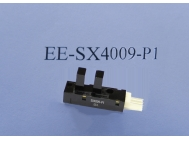• 欧姆龙激光测距传感器怎样使用？
• 本站编辑：杭州科赛控制技术有限公司发布日期：2022-03-11 16:21 浏览次数：

After the single measurement is turned on, it will enter the single measurement mode by default. Press the read key to emit laser to select the measurement point, and then press the read key to display the measurement data at the bottom of the screen.

In the mode of continuous measurement to be measured, long press the read key to enter the continuous measurement state, and the auxiliary display area of the screen will display the size peak in the continuous measurement process.

Press the area measurement button once, the screen will display a rectangle, measure the length and width according to the prompt, and the machine will automatically calculate the area.

Press the volume measurement button twice to measure the volume, measure the length, width and height according to the prompt, and the machine will automatically calculate the volume.

The Pythagorean measurement button can Pythagorean measurement, measure the bevel distance and horizontal distance according to the prompt, and automatically calculate the vertical distance; Automatically calculate the distance between the horizontal and vertical edges according to the prompt of the machine; Measure the bevel distance and horizontal distance according to the prompt, and the machine will automatically calculate the vertical distance.

The triangle area measurement button can be used for Pythagorean measurement, measure the three sides of the triangle according to the prompt, and the machine calculates the area.

The benchmark setting button can select the benchmark position, which is convenient for measurement in various environments.